• 层次聚类matlab
千次阅读
2021-04-22 03:45:37

最近需要用到层次聚类，发现在Matlab上很容易实现，下面是代码加详细注释

clear all

clc

close all

mdist=input('输入坐标文件名字\n');

disp('读取数据坐标')

%获取坐标

%文件为二维的坐标，第一列为x轴坐标，第二列为y轴坐标

%获取数据的个数存至number

[number, row]=size(xx);

%获取距离矩阵，第二参数指定距离计算方法

%可选参数有'euclidean'：欧氏距离(默认)；'seuclidean'：标准化欧氏距离；

%'mahalanobis'：马氏距离；'cityblock'：布洛克距离；'minkowski'：明可夫斯基距离；

%'cosine'：余弦距离 'correlation'：相关性 'hamming'：汉明距离 'jaccard'：Jaccard相似度

%'chebychev'：Chebychev距离。

yy=pdist(xx,'euclidean');

%获取聚类，第二参数指定层次聚类方式

%'single'：单连通，最短距离法(默认)；'complete'：全连通，最长距离法；'average'：未加权平均距离法；

%'weighted'： 加权平均法；'centroid'： 质心距离法；'median'：加权质心距离法；'ward'：内平方距离法(最小方差算法)

%指定获取簇类个数

Ncluster=input('输入类个数\n');

%获取指定Ncluster个数的聚类结果

c = cluster( zz,'maxclust', Ncluster );

%获取绘图所需颜色

%需要用到linspecer.m文件

%下载地址

%http://www.mathworks.com/matlabcentral/fileexchange/42673-beautiful-and-distinguishable-line-colors-+-colormap

if(Ncluster>12)

Color = linspecer( Ncluster );

else

Color = linspecer( Ncluster, 'qualitative' );

end

for i=1:Ncluster

for j = 1:number

if(c(j) == i)

hold on

plot(xx(j,1),xx(j,2),'o','MarkerFaceColor',Color(i,:),'MarkerEdgeColor',Color(i,:))

end

end

end

参考资料：

﻿﻿

更多相关内容
• 层次聚类matlab代码Matlab实施以下文件， “ Zhao等人，通过深度矩阵分解进行多视图聚类，AAAI'17” 摘要：由于许多现实世界的数据由不同的表示或视图组成，因此多视图聚类（MVC）最近引起了更多关注。 关键是要探索...
• 基本的层次聚类算法matlab实现 简单明了 是我以前上课时记下的笔记内容 代码在15b上实验证实可用
• 层次聚类matlab代码层次聚类算法 matlab代码，采用了单链接，完全链接和平均链接算法的层次聚类算法。
• matlab实现层次聚类法，不是用库函数实现的，而是一步步根据算法原理完成的
• 层次聚类matlab代码，数据要求字符串格式，数据类型一致，便于计算和使用，提高数据准确度和可用性，简单实用。层次聚类
• 层次聚类（hierarchical clustering）matlab算法实现：包括图，代码，数据集。
• matlab进行AHP层次分析,得出各要素的权重信息 聚类分析
•层次聚类
• 层次聚类分析,层次聚类分析热图解读,matlab源码.rar,06 层次聚类分析.m
• 使用 PHA 方法执行快速层次聚类。 该函数将从输入距离矩阵生成层次聚类树 (Z)。 输出 Z 类似于 Matlab 函数“linkage”的输出。 [主要特征] 1.比matlab联动功能更快。 2. 对混合正态分布的集群具有出色的性能。 3. ...matlab
• 层次聚类_matlab程序 标签： 层次聚类 matlab 杂谈 分类： 经典算法/数据挖掘/神经网络
• 层次聚类matlab程序，数据来源为80个平面点坐标。
• 这个是一个简单的聚类分析matlab代码实现，通过matlab对数据进行了简单的层次聚类分析matlab
• 《凝聚层次聚类算法matlab源码》由会员分享，可在线阅读，更多相关《凝聚层次聚类算法matlab源码(3页珍藏版)》请在人人文库网上搜索。1、共享一个在数据挖掘课程中作为示例使用的凝聚层次聚类算法源码，供大家学习...

《凝聚层次聚类算法matlab源码》由会员分享，可在线阅读，更多相关《凝聚层次聚类算法matlab源码(3页珍藏版)》请在人人文库网上搜索。

1、共享一个在数据挖掘课程中作为示例使用的凝聚层次聚类算法源码，供大家学习交流使用时将源码复制进一个新的Function并更改m文件的文件名为AGNES即可-2016 年4月10 日 以下为程序的 matlab 源码：function cluster=AGNES(pointSet,targetClusterNum,method)% 凝聚层次聚类算法% 输入：点集 pointSet 、目标簇数 targetClusterNum 、簇间差异度度量方式 method% 点集 pointSet为n*m矩阵，包含n个点，每个点有 m个属性% 目标簇数 targetClusterNum 为一个整数， 0tar。

2、getClusterNumn% method 为字符串，对应不同距离度量方式：% method=min:最小距离度量 ;method=max:最大距离度量 ;method=mean:均值距离度量;method=avg:平均距离度量 ;% 输出： cluster为长度为n的向量，表示各点所对应簇的类别标记% 调用方式示例：cluster=AGNES(pointSet,3,max);% 表示将 pointSet使用最大距离度量方式聚为 3 个类，将通过 cluster 变量返回类标记pointNum=size(pointSet,1);cluster=1:pointNum;%每个点对应簇标记%当前簇。

3、数目。初始默认每个点为单独的一个簇%若聚类数满足结束条件则算法结束i=0; while true i=i+1; uniCluster=unique(cluster); clusterNum=size(uniCluster,2);if clusterNum=targetClusterNum break ;end%计算簇间差异度clusterDist=zeros(clusterNum);if strcmp(method, mean )=1 %计算各簇间差异度：平均距离 clusterMean=;for c=1:clusterNum%计算各簇均值uniCluster=unique(cluster);。

4、 subCluster=pointSet(cluster=uniCluster(c),:); clusterMean=clusterMean;uniCluster(c),mean(subCluster,1);endfor d=1:size(clusterMean,1)%计算各簇间距离clusterDist(:,d)=sqrt(sum(clusterMean(:,2:end)-repmat(clusterMean(d,2:en d),size(clusterMea 门,1),1).人2,2);endclusterDist=clusterDist+eye(size(clusterDist,1)*m。

5、ax(max(clusterDist)*100; %将对角线设为最大值elseif strcmp(method, min )=1 %计算各簇间差异度：最小距离for c1=1:clusterNumfor c2=c1+1:clusterNum subPointSet1=pointSet(cluster=uniCluster(c1),:); subPointSet2=pointSet(cluster=uniCluster(c2),:); subPointSet1Expend=;for c3=1:size(subPointSet1,1) subPointSet1Expend=subPointSet1。

6、Expend;repmat(subPointSet1(c3,:),size(s ubPointSet2,1),1);end subPointSet2Expend=repmat(subPointSet2,size(subPointSet1,1),1);dist=sqrt(sum(subPoi ntSetlExpe nd-subPoi ntSet2Expe nd).A2,2); clusterDist(c1,c2)=min(dist);%取最小值endend clusterDist=clusterDist+clusterDist;%将三角阵转换为对称阵clusterDist=clusterDist。

7、+eye(size(clusterDist,1)*max(max(clusterDist)*100; %将对角线设为最大值elseif strcmp(method, max )=1 %计算各簇间差异度：最大距离for c1=1:clusterNumfor c2=c1+1:clusterNum subPointSet1=pointSet(cluster=uniCluster(c1),:); subPointSet2=pointSet(cluster=uniCluster(c2),:); subPointSet1Expend=;for c3=1:size(subPointSet1,1) subPo。

8、intSet1Expend=subPointSet1Expend;repmat(subPointSet1(c3,:),size(s ubPointSet2,1),1);endsubPointSet2Expend=repmat(subPointSet2,size(subPointSet1,1),1);dist=sqrt(sum(subPoi ntSetlExpe nd-subPoi ntSet2Expe nd).A2,2); clusterDist(c1,c2)=max(dist);%取最大值endend clusterDist=clusterDist+clusterDist;clusterDi。

9、st=clusterDist+eye(size(clusterDist,1)*max(max(clusterDist)* 100;elseif strcmp(method, avg )=1 %计算各簇间差异度：均值距离for c1=1:clusterNumfor c2=c1+1:clusterNum subPointSet1=pointSet(cluster=uniCluster(c1),:); subPointSet2=pointSet(cluster=uniCluster(c2),:); subPointSet1Expend=;for c3=1:size(subPointSet1,1) s。

10、ubPointSet1Expend=subPointSet1Expend;repmat(subPointSet1(c3,:),size(s ubPointSet2,1),1);endsubPointSet2Expend=repmat(subPointSet2,size(subPointSet1,1),1);dist=sqrt(sum(subPoi ntSetlExpe nd-subPoi ntSet2Expe nd).A2,2); clusterDist(c1,c2)=sum(dist)./size(dist,1);endend clusterDist=clusterDist+clusterDist;clusterDist=clusterDist+eye(size(clusterDist,1)*max(max(clusterDist)*100;end%合并相异度最小的两个簇rows,cols=find(clusterDist=min(min(clusterDist);两个簇index=rows,cols;%合并两个最相似的簇from=index(1,1);to=index(1,2);cluster(cluster=uniCluster(from)=uniCluster(to);end%取均值%找到相异度最小的end。

展开全文• %生成20个随机样本 %屏幕输出Q型聚类结果 %屏幕输出R型聚类结果 包含具体聚类步骤和算法，自写函数体层次聚类
• 层次聚类_matlab程序(2012-05-23 12:17:23)标签：层次聚类matlab杂谈clearclose all %清屏x1=load('数据.txt'); %加载数据x= zscore(x1); %标准化数据矩阵tic %时间监控corrdist=pdist(x,'corr'); %计算任意两点之间...

层次聚类_matlab程序

(2012-05-23 12:17:23)

标签：

层次聚类

matlab

杂谈

clear

close all %清屏

x= zscore(x1); %标准化数据矩阵

tic %时间监控

corrdist=pdist(x,'corr'); %计算任意两点之间的距离

k=聚类数；

clusters=cluster(clustertree,'maxclust',k); %聚类

c=k;

for w=1:c

[i,j]=find(clusters==w);

disp(['-----第',num2str(w),'类---------']);

disp([i,j]); %显示 i,j 值，分类结果

end

toc %时间监控结束

figure(1) %图 1

for q=1:k %分别对于每一个聚类做循环

subplot(#,#,q); %画出 q 个子图

plot(x((clusters==q),:)'); %画出所属类中所有元素曲线

axis tight

end

figure(2) %图 2

for q=1:k %分别对于每一个聚类做循环

subplot(#,#,q);

plot(mean(x((clusters==q),:))'); %画出所属类中所有元素曲线的中心曲线

axis tight

end

分享：喜欢

0赠金笔

加载中，请稍候......

评论加载中，请稍候...

发评论

登录名： 密码： 找回密码 注册记住登录状态

昵   称：

评论并转载此博文发评论

以上网友发言只代表其个人观点，不代表新浪网的观点或立场。

展开全文• 层次聚类MATLAB中实现 By:Yang Liu 1.第一种方法 （1）输入要聚类的数据。 （2）计算各个样本之间的欧氏距离。 （3）把距离化成矩阵，矩阵中的元素Xij{X_{ij}}Xij​表示第i个样本和第j个样本的距离。 （4）生成...

## 层次聚类在MATLAB中实现

By:Yang Liu
1.第一种方法
（1）输入要聚类的数据。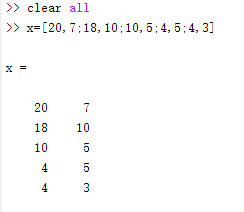（2）计算各个样本之间的欧氏距离。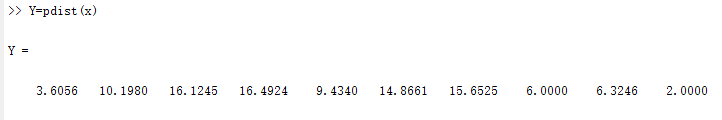（3）把距离化成矩阵，矩阵中的元素 X i j {X_{ij}} 表示第i个样本和第j个样本的距离。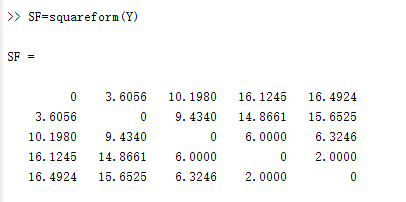（4）生成聚类数矩阵，前两列表示是第几簇，最后一列表示两个簇的距离，原样本一共5个元素，所以初始有5簇，则当完成一次聚类后，新得到的簇，就是第六簇（此例中第六簇就是第四簇和第五簇合并产生），以此类推。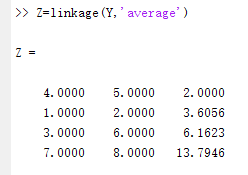（5）生成可视化树状图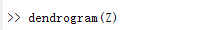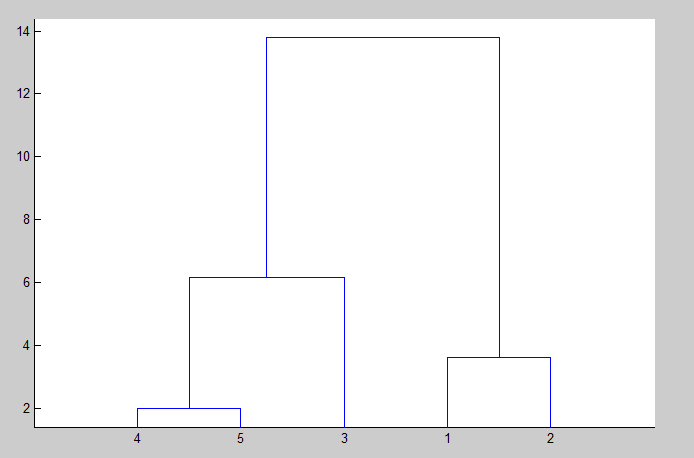（6）按需求划分，例中为划分三簇，T为各个样本划分到第几簇的结果。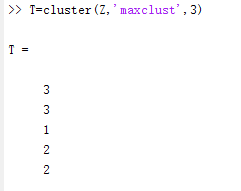2.第二种方法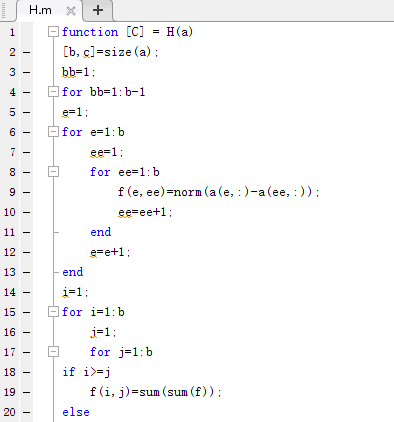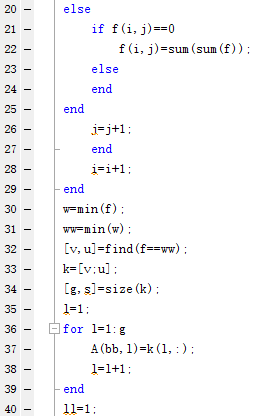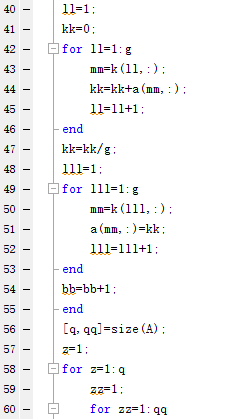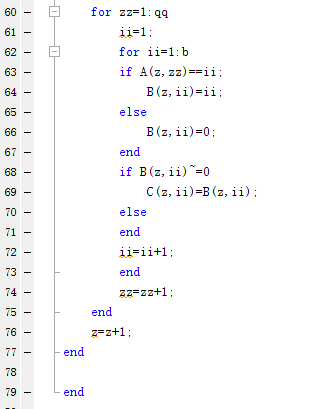测试结果：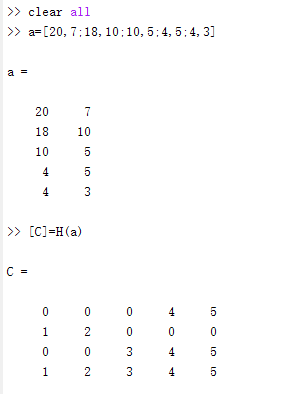结果矩阵C含义：第一次把第四和第五样本分成一簇；第二次把第一和第二样本分成一簇；第三次把第三四五样本分成一簇，第四次把所有样本分成一簇。
若要把样本分成N簇，则查看倒数第N行。
假设要把样本分成三簇，则通过观察倒数第三行可知第四个和第五个样本为一簇，第一个和第二个样本为一簇，第三个样本为一簇，最终结果与第一种方法所得结果完全一致。
参考文献：
（1）https://blog.csdn.net/henu111/article/details/81512314
（2）https://blog.csdn.net/qq278672818/article/details/62038527

展开全文聚类算法 matlab 机器学习
• 聚类分析 | MATLAB实现HC(层次聚类)分析 目录聚类分析 | MATLAB实现HC(层次聚类)分析层次聚类简介数据下载程序设计学习小结参考资料致谢 层次聚类简介 层次聚类是通过创建聚类树，同时在多个距离尺度内调查数据分组...聚类分析 层次聚类 机器学习
• 使用MATLAB进行K-means聚类和层次聚类matlab
• 本专栏第二篇文章介绍过层次聚类法 数学建模学习笔记（二）层次聚类matlab代码如下： clc; clear; Y=[0.080 0.143 2.000 0.250 0.500 0.286 0.143 2.000 2.000 inf]; Z=linkage(Y,'average') dendrogram(Z) 然而...
• 一、层次聚类介绍1.1 简介层次聚类，主要是对给定的待聚类的数据集进行层次化分解。主要分为两大类：1.从下到上的凝聚聚类2.从上到下的分裂聚类其他算法大部分是对样本之间距离度量或者类间凝聚/分裂依据进行改进。...
• 层次聚类(Hierarchical Clustering)是聚类算法的一种，通过计算不同类别数据点间的相似度来创建一棵有层次的嵌套聚类树。k-means聚类对于下面这种文章略显鸡肋 这种情况考虑用层次聚类发法就比较好了。程序相关的...
• ## matlab层次聚类

万次阅读 多人点赞 2018-12-10 20:07:11层次聚类
• ## （MATLAB）层次聚类

千次阅读 2020-02-02 16:05:26
dendrogram(Z) %可视化层次聚类树 Z %Z是一个(m-1)*3的矩阵,Z数组的前两列是索引下标列，最后一列是距离列 效果图如下： 其中，横坐标为index，纵坐标为距离。比如，图中2号和4号的距离为1.0228。 打印...matlab...